The OpenFOAM Foundation
targetCoeffTrim Class Reference

Target trim forces/coefficients. More...

Inheritance diagram for targetCoeffTrim: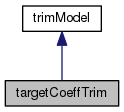[legend]
Collaboration diagram for targetCoeffTrim: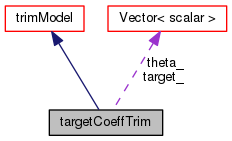[legend]

## Public Member Functions

TypeName ("targetCoeffTrim")
Run-time type information. More...

targetCoeffTrim (const fv::rotorDiskSource &rotor, const dictionary &dict)
Constructor. More...

virtual ~targetCoeffTrim ()
Destructor. More...

void read (const dictionary &dict)

virtual tmp< scalarFieldthetag () const
Return the geometric angle of attack [rad]. More...

virtual void correct (const vectorField &U, vectorField &force)
Correct the model. More...

virtual void correct (const volScalarField rho, const vectorField &U, vectorField &force)
Correct the model for compressible flow. More...

template<class RhoFieldType >
Foam::vector calcCoeffs (const RhoFieldType &rho, const vectorField &U, const scalarField &thetag, vectorField &force) constPublic Member Functions inherited from trimModel
TypeName ("trimModel")
Run-time type information. More...

declareRunTimeSelectionTable (autoPtr, trimModel, dictionary,(const fv::rotorDiskSource &rotor, const dictionary &dict),(rotor, dict))

trimModel (const fv::rotorDiskSource &rotor, const dictionary &dict, const word &name)
Construct from components. More...

virtual ~trimModel ()
Destructor. More...

## Protected Member Functions

template<class RhoFieldType >
vector calcCoeffs (const RhoFieldType &rho, const vectorField &U, const scalarField &alphag, vectorField &force) const
Calculate the rotor force and moment coefficients vector. More...

template<class RhoFieldType >
void correctTrim (const RhoFieldType &rho, const vectorField &U, vectorField &force)
Correct the model. More...

## Protected Attributes

label calcFrequency_
Number of iterations between calls to 'correct'. More...

bool useCoeffs_
Flag to indicate whether to solve coeffs (true) or forces (false) More...

vector target_
Target coefficient vector (thrust force, roll moment, pitch moment) More...

vector theta_
Pitch angles (collective, roll, pitch) [rad]. More...

label nIter_
Maximum number of iterations in trim routine. More...

scalar tol_
Convergence tolerance. More...

scalar relax_
Under-relaxation coefficient. More...

scalar dTheta_
Perturbation angle used to determine jacobian. More...

scalar alpha_
Coefficient to allow for conversion between US and EU definitions. More...Protected Attributes inherited from trimModel
const fv::rotorDiskSourcerotor_
Reference to the rotor source model. More...

const word name_
Name of model. More...

dictionary coeffs_
Coefficients dictionary. More...

## Additional Inherited MembersStatic Public Member Functions inherited from trimModel
static autoPtr< trimModelNew (const fv::rotorDiskSource &rotor, const dictionary &dict)
Return a reference to the selected trim model. More...

## Detailed Description

Target trim forces/coefficients.

Solves:

c^old + J.d(theta) = c^target

Where:

n = time level c = coefficient vector (thrust force, roll moment, pitch moment) theta = pitch angle vector (collective, roll, pitch) J = Jacobian [3x3] matrix

The trimmed pitch angles are found via solving the above with a Newton-Raphson iterative method. The solver tolerance can be user-input, using the 'tol' entry.

If coefficients are requested (useCoeffs = true), the force and moments are normalised using:

force c = ——————————— alpha*pi*rho*(omega^2)*(radius^4)

and

moment c = ——————————— alpha*pi*rho*(omega^2)*(radius^5)

Where:

alpha = user-input conversion coefficient (default = 1) rho = desity omega = rotor angulr velocity pi = mathematical pi

Source files

Definition at line 87 of file targetCoeffTrim.H.

## ◆ targetCoeffTrim()

 targetCoeffTrim ( const fv::rotorDiskSource & rotor, const dictionary & dict )

Constructor.

Definition at line 194 of file targetCoeffTrim.C.

Referenced by targetCoeffTrim::correctTrim().

Here is the call graph for this function: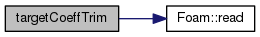Here is the caller graph for this function: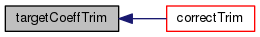## ◆ ~targetCoeffTrim()

 ~targetCoeffTrim ( )
virtual

Destructor.

Definition at line 216 of file targetCoeffTrim.C.

## ◆ calcCoeffs() [1/2]

 vector calcCoeffs ( const RhoFieldType & rho, const vectorField & U, const scalarField & alphag, vectorField & force ) const
protected

Calculate the rotor force and moment coefficients vector.

## ◆ correctTrim()

 void correctTrim ( const RhoFieldType & rho, const vectorField & U, vectorField & force )
protected

Correct the model.

Definition at line 100 of file targetCoeffTrim.C.

Referenced by targetCoeffTrim::calcCoeffs().

Here is the call graph for this function: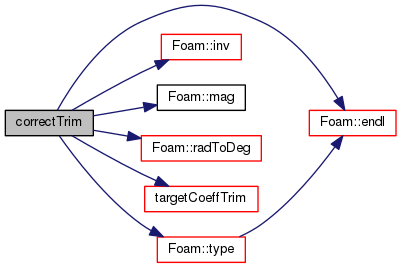Here is the caller graph for this function: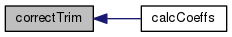## ◆ TypeName()

 TypeName ( "targetCoeffTrim" )

Run-time type information.

 void read ( const dictionary & dict )
virtual

Reimplemented from trimModel.

Definition at line 222 of file targetCoeffTrim.C.

Here is the call graph for this function: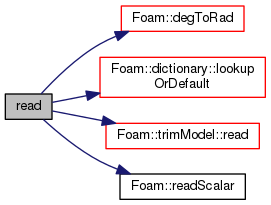## ◆ thetag()

 Foam::tmp< Foam::scalarField > thetag ( ) const
virtual

Return the geometric angle of attack [rad].

Implements trimModel.

Definition at line 258 of file targetCoeffTrim.C.

Here is the call graph for this function: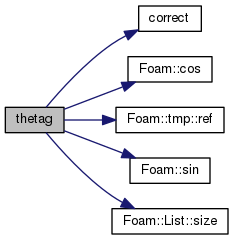## ◆ correct() [1/2]

 void correct ( const vectorField & U, vectorField & force )
virtual

Correct the model.

Implements trimModel.

Definition at line 276 of file targetCoeffTrim.C.

Referenced by targetCoeffTrim::thetag().

Here is the caller graph for this function: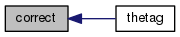## ◆ correct() [2/2]

 void correct ( const volScalarField rho, const vectorField & U, vectorField & force )
virtual

Correct the model for compressible flow.

Implements trimModel.

Definition at line 286 of file targetCoeffTrim.C.

## ◆ calcCoeffs() [2/2]

 Foam::vector calcCoeffs ( const RhoFieldType & rho, const vectorField & U, const scalarField & thetag, vectorField & force ) const

Definition at line 46 of file targetCoeffTrim.C.

Here is the call graph for this function: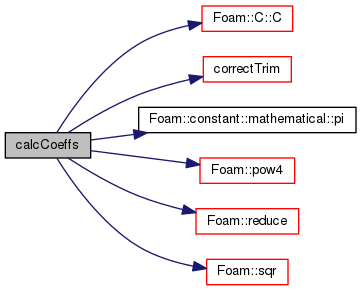## ◆ calcFrequency_

 label calcFrequency_
protected

Number of iterations between calls to 'correct'.

Definition at line 97 of file targetCoeffTrim.H.

## ◆ useCoeffs_

 bool useCoeffs_
protected

Flag to indicate whether to solve coeffs (true) or forces (false)

Definition at line 100 of file targetCoeffTrim.H.

## ◆ target_

 vector target_
protected

Target coefficient vector (thrust force, roll moment, pitch moment)

Definition at line 103 of file targetCoeffTrim.H.

## ◆ theta_

 vector theta_
protected

Pitch angles (collective, roll, pitch) [rad].

Definition at line 106 of file targetCoeffTrim.H.

## ◆ nIter_

 label nIter_
protected

Maximum number of iterations in trim routine.

Definition at line 109 of file targetCoeffTrim.H.

## ◆ tol_

 scalar tol_
protected

Convergence tolerance.

Definition at line 112 of file targetCoeffTrim.H.

## ◆ relax_

 scalar relax_
protected

Under-relaxation coefficient.

Definition at line 115 of file targetCoeffTrim.H.

## ◆ dTheta_

 scalar dTheta_
protected

Perturbation angle used to determine jacobian.

Definition at line 118 of file targetCoeffTrim.H.

## ◆ alpha_

 scalar alpha_
protected

Coefficient to allow for conversion between US and EU definitions.

Definition at line 121 of file targetCoeffTrim.H.

The documentation for this class was generated from the following files:
• src/fvOptions/sources/derived/rotorDiskSource/trimModel/targetCoeff/targetCoeffTrim.H
• src/fvOptions/sources/derived/rotorDiskSource/trimModel/targetCoeff/targetCoeffTrim.C